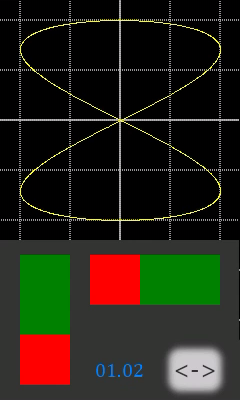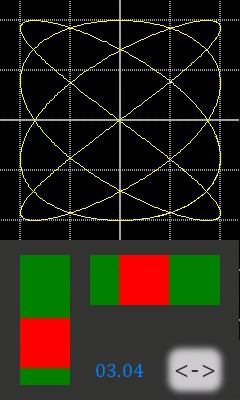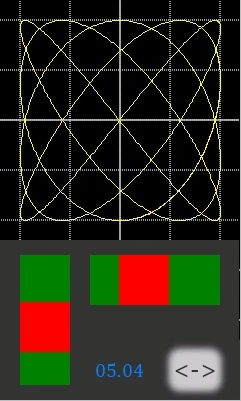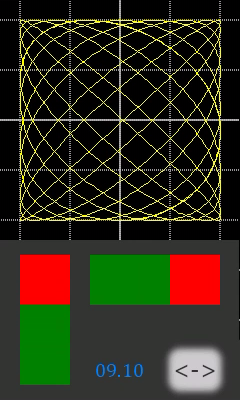## The Sunday blog: Boost your HMI with advanced mathematics!

### Part 5: Harmonic motion

In the last episode (Part 4: Trigonometry), we have learned how to quickly and precisely iterate through a full period of sin/cos values, which allowed us to draw either circles having identical radii in x and y direction, or ellipses having different x and y radii. It’s time to move on! The French physicist Jules Antoine Lissajous wondered during the 19th century how a geometric figure would look like if one didn’t vary the radii in x and y direction as we did when drawing the ellipses, but if one had moving the circular line with different speeds or frequencies, depending on the direction. Mr Lissajous used a complex setting with two tuning forks (set on different tone heights) with a small mirror attached to each, one deflecting a light beam vertically, and the other horizontally. That’s how he discovered very beautiful patterns which where later called Lissajous curves.

#### Some little mathematics

Remember last week. Drawing a circle was done by two very simple parametric equations:

`y = r * cos( m ) and x = r * sin( m )`

and the ellipses were only slightly more complex:

`y = r_y * cos( m ) and x = r_x * sin( m )`

For our Lissajous curves, the radius will again be the same in both directions, but the periodicity will be different:

`y = r * cos( m_y ) and x = r * sin( m_x )`

Since using our iterative algorithm requires synchronous stepping through the sine and cosine values, we will need two sin/cos pairs today, step through these at different speeds (step sizes) and take the cos value from one and the sin value from the other into account for drawing.

#### Keep it dynamic!

A simple and Nextion-like way to vary the complexity of our Lissajous curves is the use of sliders. There is no fun in having identical m_y and m_x values (you guessed it by looking at the equations above, we’d only see a circle), so we use some little trickery which multiplies the vertical slider (h0) value from 1 to 5 by 2 before subtracting 1, so that we get the values 1, 3, 5, 7 and 9 for m_y. The values from the horizontal slider (h1) are just multiplied by 2, so that we can have 2, 4, 6, 8 or 10 for m_x. As a visual feedback, we added a float component with two digits on the right side and we update it before each drawing by 100 * m_y + m_x, so that it will always indicate us m_y.m_x

#### The code

As usual, we define all required variables and constants in the program.s tab:

```//The following code is only run once when power on, and is generally used for global variable definition and power on initialization data
int sys0=0,sys1=0,sys2=0 //At present, the definition of global variable only supports 4-byte signed integer (int), and other types of global quantity declaration are not supported. If you want to use string type, you can use variable control in the page to implement
//Trigonometry variables
int sin1,cos1,sin2,cos2
//Coordinates, drawing and transformation:
int amp=-100
int x_c=120
int y_c=120
int c_steps=1257
int twopi=205783 //This is an approximated value for 2*PI in q15
int round=16384 //This is 0.5 in q15
int c_coeff
int x,x_last,y,y_last,k
int my=1,mx=2
page 0 //Power on start page 0```

Then, we handle the vertical slider action in the Touch Release event:

```my=h0.val*2-1 //Transform 1,2,3,4,5 into 1,3,5,7,9
click m0,0 //Start drawing```

Similar for the horizontal slider:

```mx=h1.val*2 //Transform 1,2,3,4,5 into 2,4,6,8,10
click m0,0 //Start drawing```

And finally the iterative calculation and drawing routine, in the Touch Release event of hotspot m0. Without the comments, there are only 26 lines of active code:

```//Clear screen and set initial condition
ref page0
x0.val=100*my+mx //Display the y/x ratio
sin1=0
sin2=0
cos1=32768 //This is 1 in q15
cos2=32768
x_last=sin1*amp>>15+x_c
y_last=cos2*amp>>15+y_c
c_coeff=twopi+round/c_steps
for(k=1;k<=c_steps;k++)
{
//Calculate new sin and cos values
sys0=c_coeff*cos1*my+round>>15
sin1+=sys0
sys0=c_coeff*cos2*mx+round>>15
sin2+=sys0
sys0=c_coeff*sin1*my+round>>15
cos1-=sys0
sys0=c_coeff*sin2*mx+round>>15
cos2-=sys0
//Draw shape
x=-1*sin2*amp+round>>15+x_c
y=-1*cos1*amp+round>>15+y_c
line x_last,y_last,x,y,YELLOW
x_last=x
y_last=y
doevents // <- Remove this for quicker drawing
}```

#### The result

I’ll let some pictures talk. Feel free (as always) to download the full HMI file from the Nextion forums to play and experiment with!#### Next week: It will be your turn!

NEXTION will organize a contest: In next week’s Sunday blog, you will see a short video showing again a different class of periodical curves.

You will have to use your knowledge acquired through these 5 advanced mathematics blog episodes to write the corresponding Nextion code. The most efficient, quick and precise solution, sent in as a working HMI file, will win a price. All details next Sunday!